GFG App
Open AppBrowser
Continue

# MATLAB – Texture Measures from GLCM

GLCM stands for Gray Level Co-occurrence Matrix. In image processing, The GLCM function computes how often pairs of pixels with a particular value and in a particular spatial relationship occur in an image, constructs a GLCM, and extracts statistical measures from this matrix to determine the texture of an image. characterize the (The texture filter functions described in Computing Statistical Texture Measures cannot provide information about the shape, that is, the spatial relationship of pixels in an image.)

## Texture Analysis in MATLAB

Texture analysis refers to characterizing regions in an image by their texture content. Texture analysis attempts to quantify intuitive qualities described by terms such as rough, smooth, silky, or bumpy as a function of spatial variation in pixel intensity. In this sense, roughness or non-uniformity refers to variations in intensity values ​​or gray levels.

Texture Analysis defines some functionality as follows.

• entropy – it’s a statistical measure of randomness.
• entropyfilt – it’s used to measure some relative changes in the image.
• rangefilt – it’s can return an array of output pixels.
• graycomatrix – it’s used to calculate the GLCM form of an image.
• graycoprops – it can normalize the gray-level co-occurrence matrix (GLCM).

## Calculation of Statistical Measure Texture

A texture analysis function that filters an image using standard statistical means. These statistics provide information about the local variability of the intensity values ​​of pixels in the image, thus allowing us to characterize the texture of the image.

Syntax

E = entropyfilt(I)

% output pixels contains the entropy values of pixels in input image I.

E = entropyfilt(I, node)

% it is perform entropy filtering using the neighborhood node.

## Stepwise Implementation Texture Measures from GLCM

Step 1:  So, first of all, we plot the GFG image in MATLAB figure using imshow() function using the following line of MATLAB code in MATLAB editor.

## Matlab

 `% Code for represent image in figure.` `clc` `clear all;` `close all;` `warning off` `I = imread(``'GFG.png'``);  ``% Read the image ` `imshow(I);     ``% plotting the image in figure.` `title(``'Original Image'``);`

Output: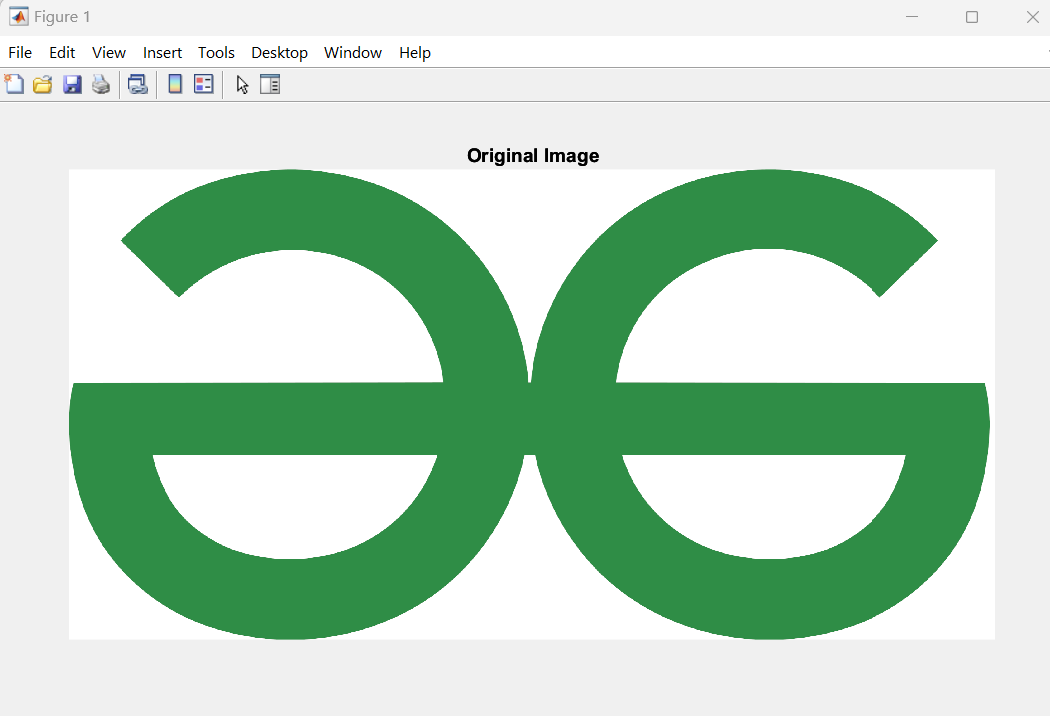Step 2:  Now, in this step, we will compute the entropy using the entropyfilt. following line of code.

## Matlab

 `% Code for represent image in figure.` `clc` `clear all;` `close all;` `warning off` `I = imread(``'GFG.png'``);  ``% Read the image ` `imshow(I);     ``% plotting the image in figure.` `title(``'Original Image'``);` `E = entropyfilt(I);` `figure;` `imshow(E);` `title(``'Result of Entropy Filtering'``);`

After running the above code you will get entropy filtered image in figure 2.

Output: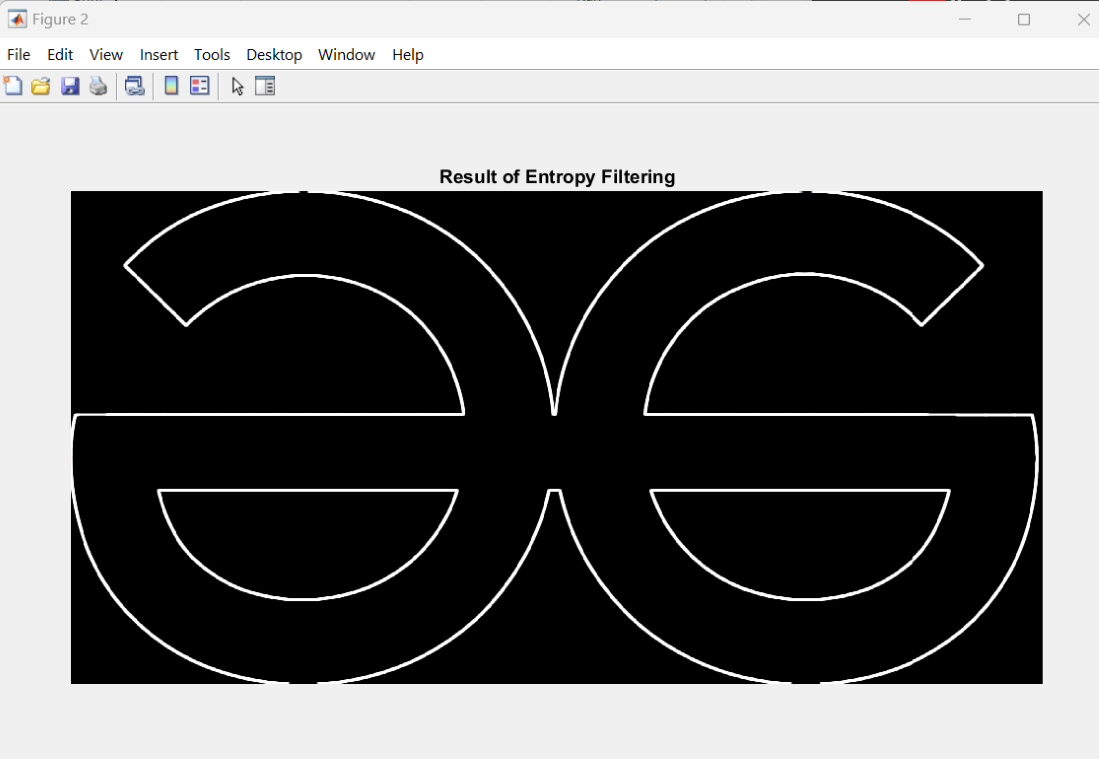So in this above output why the entropy is showing a white line because of that the entropyfilt is returned high-intensity values. so to check the entropyfilt value we have to enter the command in MATLAB Command Window, as you can see below.

>> max(max(E))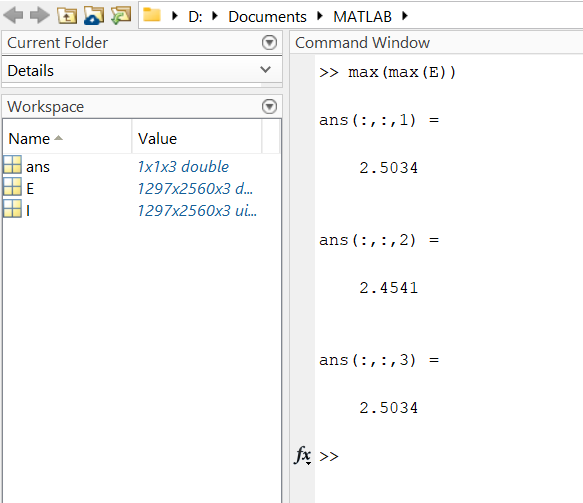Step 3: Now in this step we will just normalize the entropyfilt image output by implementing just one line of code in the previous code.

## Matlab

 `% Code` `E = rescale(entropyfilt(I));` `figure;` `imshow(E);` `title(``'Result of rescale(Entropy Filtering)'``);`

Output: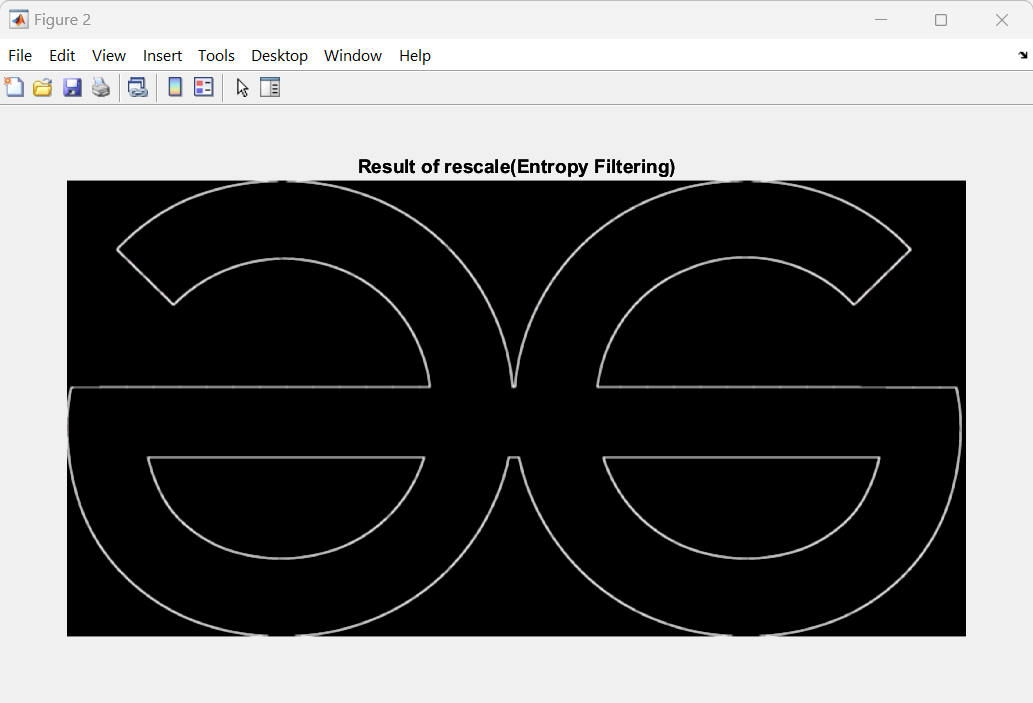As you can see the implemented output is rescaled and shown in figure2, in this output the outline is a little bit changed compared to the entropyfilt output. and the image pixels are light regions.

Step 4: Now, what we have to do with the entropyfilt is used to segmentation of Texture. now we will apply the thresholding for getting the proper threshold value we will plot the histogram.

For Differentiate the threshold value from the plot we will apply the trial and error method. using the following line of code.

## Matlab

 `% Code` `figure;` `imhist(E);` `binary_img = imbinarize(E,0.7);` `figure;` `imshow(binary_img);`

Output: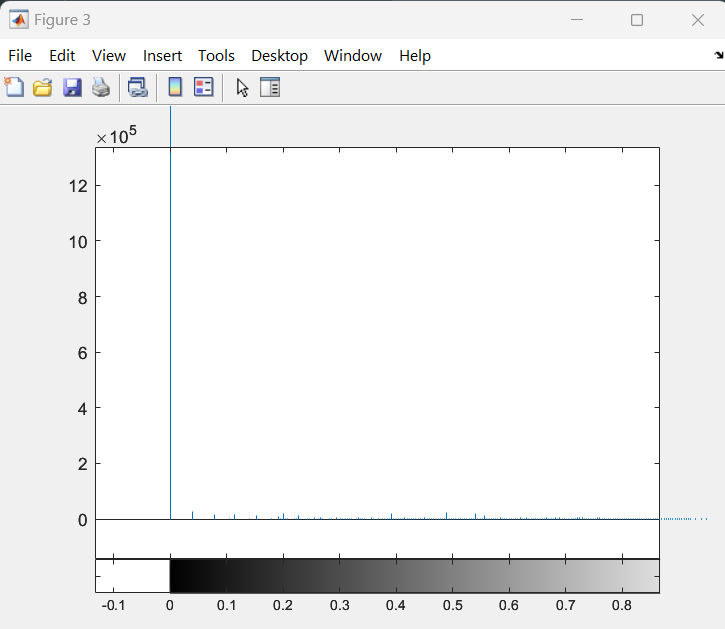## Matlab

 `% Code for represent image in figure.` `clc` `clear all;` `close all;` `warning off` `I = imread(``'GFG.png'``);  ``% Read the image ` `imshow(I);     ``% plotting the image in figure.` `title(``'Original Image'``);` `E = rescale(entropyfilt(I));` `figure;` `imshow(E);` `title(``'Result of rescale(Entropy Filtering)'``);` `figure;` `imhist(E); ``% plotting the histogram of the rescaled image.` `binary_img = imbinarize(E,0.7);` `figure;` `imshow(binary_img); ``% present the binary image of histogram.` `figure;` `area_opened = bwareaopen(binary_img,1000);` `imshow(area_opened)`

After completing the implementation code we will apply the trial-and-run method and get the final output according to the implementation code. The final output comes in figure4 as a blank graph(figure) because of the thresholding values with the (Texture Measures from GLCM)  binary image which is not showing in the graph.

Output :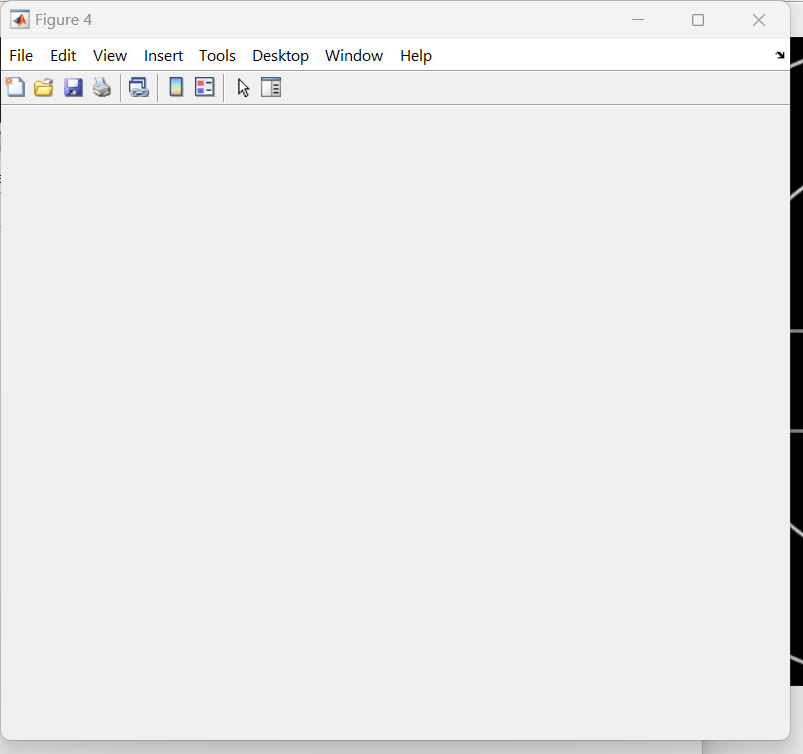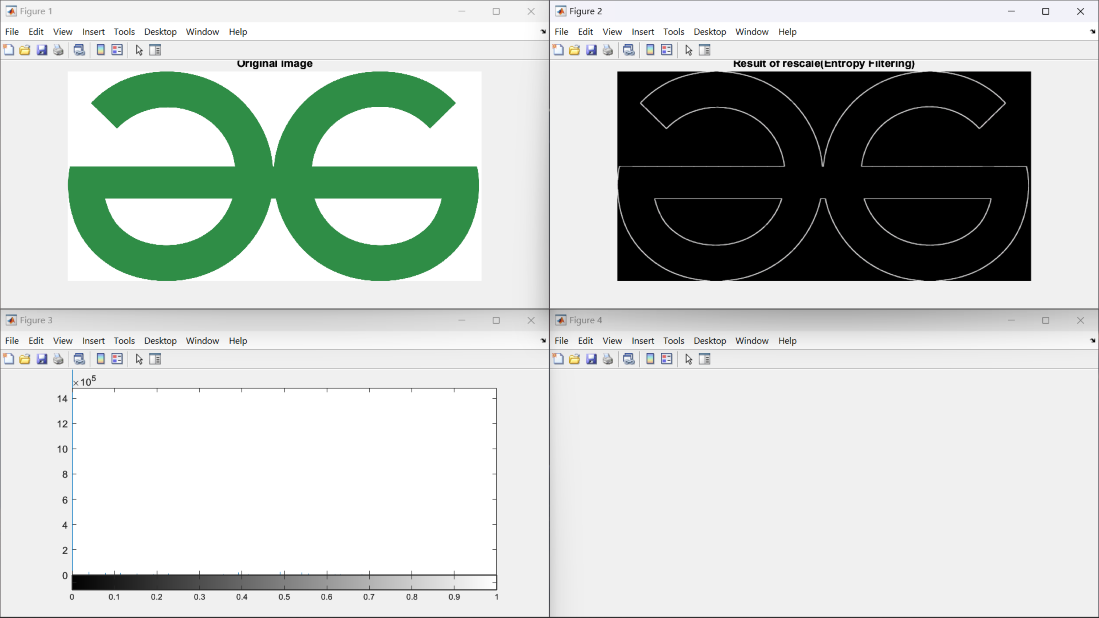So, using the entropyfilt we can Texture Measures from GLCM(Gray Level Co-occurrence Matrix) technique to differentiate the different types of intensity level values in the images.

My Personal Notes arrow_drop_up